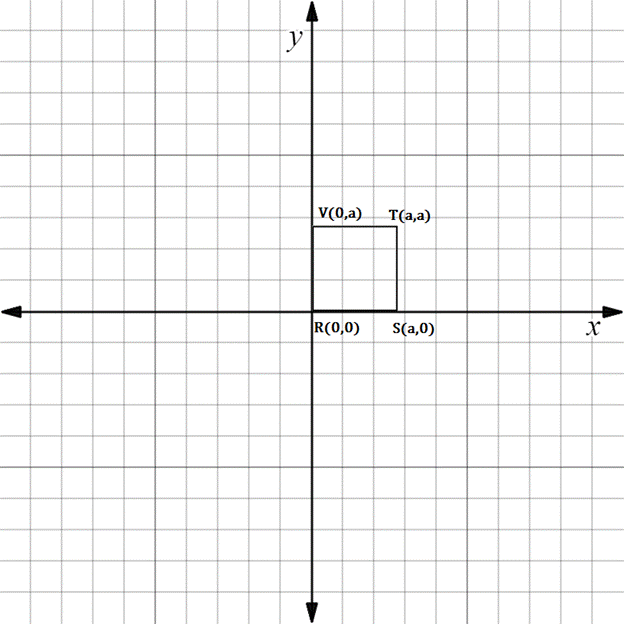Chapter 10.3, Problem 10EElementary Geometry For College St...

7th Edition
Alexander + 2 others
ISBN: 9781337614085

Solutions

Chapter
SectionElementary Geometry For College St...

7th Edition
Alexander + 2 others
ISBN: 9781337614085
Textbook Problem

In Exercises 5 to 10, the real numbers a, b, c, and d are positive.Consider the quadrilateral with vertices at R 0 ,   0 , S a ,   0 , T a ,   a , and V 0 ,   a . Explain why R S T V is a square.To determine

To explain:

RSTV is a square.

Explanation

The given diagram is shown below.

To prove RSTV is a square, we have to prove the following statement.

All sides are equal and parallel.

Now, we have to find the whether opposite sides are equal.

The distance between MN is equal to the distance between QP.

The distance between MQ is equal to the distance between NP.

Find the length of the side RS.

x1,y1=R0, 0

x2,y2=Sa, 0

Formula for distance between two points

dRS=x2-x12+y2-y12

Substituting the x and y co-ordinates

dRS=a-02+0-02

On solving this,

dRS=a2+02

dRS=a2

dRS=a

Then, find the length of the side ST.

x1,y1=Sa,0

x2,y2=Ta, a

Substituting the x and y co-ordinates

dST=a-a2+a-02

On solving this,

dST=02+a2

dST=a2

dST=a

Find the length of the side TV.

x1,y1=Ta, a

x2,y2=V0, a

Substituting the x and y co-ordinates

dTV=0-a2+a-a2

On solving this,

dTV=-a2+02

dTV=a2

dTV=a

Then, find the length of the side VR.

x1,y1=V0, a

x2,y2=R0, 0

Substituting the x and y co-ordinates

dVR=0-02+0-a2

On solving this,

dVR=02+-a2

dVR=a2

dRS=dST=dTV=dVR

Hence, the all the sides are equal

Still sussing out bartleby?

Check out a sample textbook solution.

See a sample solution

The Solution to Your Study Problems

Bartleby provides explanations to thousands of textbook problems written by our experts, many with advanced degrees!

Get Started

Find more solutions based on key concepts